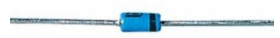﻿ What is a Schottky Diode? ﻿# What is a Schottky Diode?A schottky diode is a diode that has a low forward voltage drop and a very fast switching ability.

Therefore, they are used in applications where a circuit needs to function off of a very low voltage and in all types of high-speed switching applications such as in generator, detectors, and RF applications.

### Construction

A shottky diode has a number of superior characteristics than a standard diode because it is constructed different.

A conventional diode is composed of a PN junction. This PN junction forms a depletion layer in between the P and N material. This depletion layer creates a barrier between these n and p materials. Because of this, the PN junction will need a strong voltage to push the electrons across to the holes, so that current flows. This is how current flow is achieved in typical diodes.

A schottky diode, however, is made from a metal semiconductor junction. This metallic junction, which is more conductive, allows for easier conduction across than with a typical PN junction. Therefore, not as strong a voltage is needed to create current flow across the junction. Therefore, the threshold voltage needed to create large current across the junction is smaller. This is what allows the diode to have a very low forward voltage drop and a faster switching speed.

### Low Forward Voltage Drop

One of the chief characteristics schottky diodes are known for are their low forward voltage drops.

Their low forward voltage drops means that they consume less voltage than a typical diode.

A typical silicon diode consumes about 0.7V across its junction.

A typical schottky diode may consume only 0.3-0.4V across its junctions. Thus, it saves about 300mV of power.

Below is a chart of a schottky's diode forward voltage vs. forward current characteristics:You can see that with a forward voltage dropped across the diode between 0.3V and 0.4V, the schottky diode's current begins to significantly increase. For a typical diode again, this value would not be so low. A typical diode would need between 0.6V and 0.7V in order to significantly increase the current. So, again, schottky diode's consume less power than most other diodes. For this reason, they are used for low-voltage applications, such as when a circuit needs to operate using a very low amount of voltage.

To illustrate this and demonstrate this in an actual circuit, let's look at the following 2 circuits shown below:In the circuits above, one contains a conventional silicon diode and the other contains a schottky diode. Both circuits are supplied with power from a 2V DC power source.

Using this as an example, we can show how power is allocated more efficiently with a schottky diode rather than a conventional one.

You can see that the conventional silicon diode consumes a voltage drop of 0.7V. Therefore, there is 1.3V remaining to be dropped across the load that has to be powered. The schottky diode, however, consumes a voltage drop of approximately 0.3V. Therefore, there is 1.7V remaining to be dropped across the load. If the load needs 1.5V in ordered to be turned on, the conventional silicon diode could not achieve this. Either more voltage would have to be supplied to the circuit, or the load remain off. With circuits that have power constraints and must run off of low power, the schottky diode is much more efficient in saving power, since it uses less power.

These are practical circuit examples to see the power efficiency of schottky diodes.

### Fast Switching

Schottky diodes are also characterized by their extremely fast switching ability.

Because of this, they are used as ultrafast switches in circuits. For this reason, they are useful in VHF (Very High Frequency) and UHF (Ultra High Frequency) circuits. They are used as switching elements in generators, detectors, motor driver circuits, RF circuits, and a variety of other circuits.